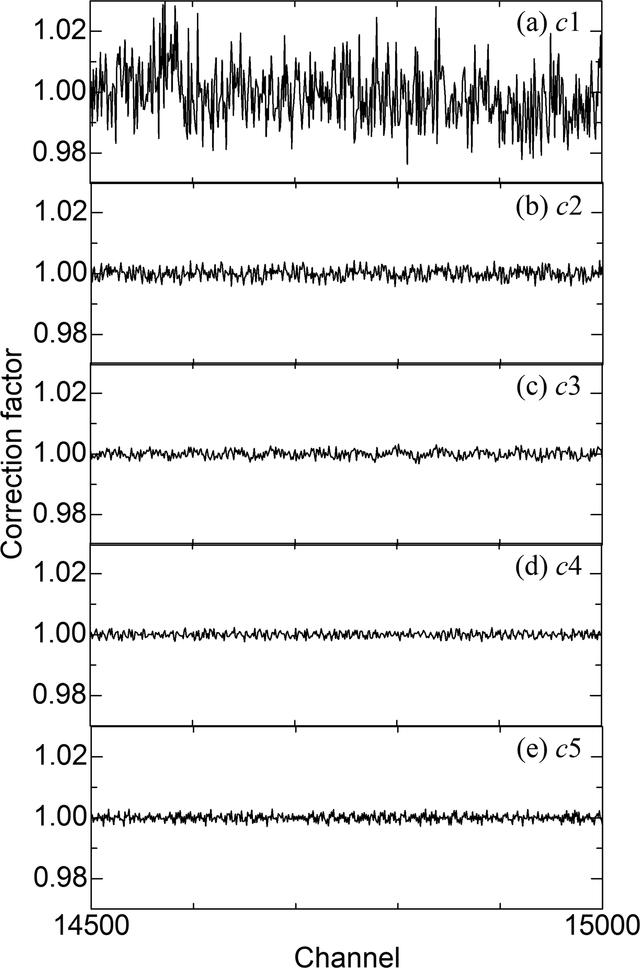disable zoom     view article Figure 3 Correction factors from channels 14500 to 15000 at each step in the multi-step process: (a) the first-step factors c1 in equation (4), (b) the second-step factors c2 in equation (6), (c) the third-step factors c3 in equation (8), (d) the fourth-step factors c4 in equation (10) and (e) the fifth-step factors c5 in equation (12).JOURNAL OFSYNCHROTRONRADIATION
ISSN: 1600-5775
Volume 26| Part 3| May 2019| Pages 762-773